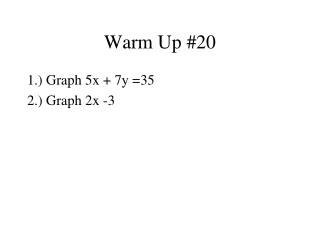DownloadDownload PresentationWarm Up #20

# Warm Up #20

Download Presentation## Warm Up #20

- - - - - - - - - - - - - - - - - - - - - - - - - - - E N D - - - - - - - - - - - - - - - - - - - - - - - - - - -
##### Presentation Transcript

1. Warm Up #20 1.) Graph 5x + 7y =35 2.) Graph 2x -3

2. Graph 5x + 7y =35 Solve for “y” 7y = -5x +35 Y = -5/7 x + 5

3. Find 3 points using the table, and graph the line of the equation.y = 2x - 3 1 -1 0 -3 -5 -1

4. 7.3 Linear Equations and Their Graphs Objective: • To graph linear equations using the x and y intercepts • To graph horizontal and vertical lines

5. Linear Equations(Graphs are straight lines) • Equation is linear only if the each variable has an exponent of “1”. • (exponent in denominator is not linear) • Products of variables not linear, ie (x)(y) y – 3x = -7 y = 2x + 1 y = x2 + 1 5y = 14 xy = 2

6. y x - intercept X y - intercept

7. Graphing using Intercepts Let x=0 and determine the y-intercept. Let y=0 and determine the x-intercept Plot both points. Connect them with a line.

8. Graph 4x + 3y = 12 using intercepts Find x-intercept 4x + 3(0) = 12 Find y-intercept 4(0) + 3y = 12 4x = 12 3y = 12 x = 3 y = 4

9. Horizontal and Vertical Lines • The graph of y= # is HORIZONTAL • The graph x =# is VERTICAL

10. Graph 4y = 16 using 3-points

11. Graph 3x = 18 using 3-points

12. Differences between graphing by using a table and graphing by finding the x and y intercepts • When graphing by a table you need to solve for y (Slope Intercept Form y=mx+b) • When graphing by finding the x and y intercepts you do not have to solve for y (Standard Form Ax +By =C)

13. AssignmentPage 316 16 – 40 (even)(use graph paper)